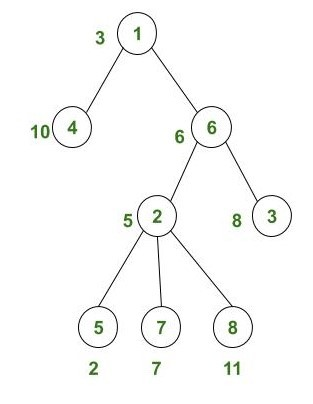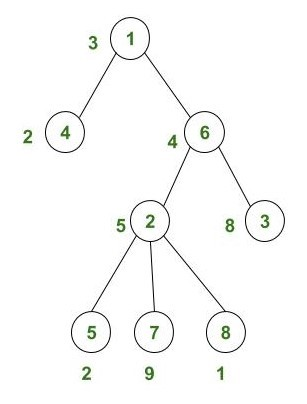Related Articles

# Minimum difference between any two weighted nodes in Sum Tree of the given Tree

• Difficulty Level : Expert
• Last Updated : 13 Aug, 2021

Given a tree of N nodes, the task is to convert the given tree to its Sum Tree(including its own weight) and find the minimum difference between any two node’s weight of the sum tree.

Note: The N nodes of the given tree are given in the form of top to bottom with N-1 line where each line describes two nodes that are connected.

Examples:

Input:Output: 1
Explanation:
total weight of node 1: 3 (own weight) + (10 + 6 + 5 + 8 + 2 + 7 + 11) (sub-tree node’s weight) = 52
total weight of node 2: 5 (own weight) + (2 + 7 + 11) (sub-tree node’s weight) = 25
total weight of node 3: 8 (own weight) + (0) (sub-tree node’s weight) = 8
total weight of node 4: 10 (own weight) + (0) (sub-tree node’s weight) = 10
total weight of node 5: 2 (own weight) + (0) (sub-tree node’s weight) = 2
total weight of node 6: 6 (own weight) + (5 + 8 + 2 + 7 + 11) (sub-tree node’s weight) = 39
total weight of node 7: 7 (own weight) + (0) (sub-tree node’s weight) = 7
total weight of node 8: 11 (own weight) + (0) (sub-tree node’s weight) = 11
By observing the total weight of each node, Node 4 and 8 have a minimum difference(11-10) = 1

Input:Output: 0

Approach:

1. We will traverse the given tree from below and store the weight of that node plus its sub-tree node’s weight in one array and mark the index of each node as visited. So in between, if we revisit that node then we don’t have to count the weight of that node again.
2. We will sort the array where we have stored the total weight of each node.
3. Now find the pairwise difference in the sorted array and whichever pair gave minimum difference print that minimum difference at last.

Below is the implementation of the above approach:

## C++

 `// C++ program for the above approach` `#include ``using` `namespace` `std;` `// Function to find minimum``// difference between any two node``void` `MinimumDifference(``int` `total_weight[],``                       ``int` `N)``{``    ``int` `min_difference = INT_MAX;` `    ``for` `(``int` `i = 1; i < N; i++) {` `        ``// Pairwise difference``        ``if` `(total_weight[i]``                ``- total_weight[i - 1]``            ``< min_difference) {``            ``min_difference``                ``= total_weight[i]``                  ``- total_weight[i - 1];``        ``}``    ``}` `    ``cout << min_difference << endl;``}` `// Function to find total weight``// of each individual node``void` `SumTree(vector > v,``             ``int` `individual_weight[],``             ``int` `N)``{``    ``// Array to store total weight``    ``// of each node from 1 to N``    ``int` `total_weight[N] = { 0 };` `    ``// Array to keep track of node``    ``// previously counted or not``    ``int` `visited[N] = { 0 };` `    ``// To store node no. from``    ``/// N-1 lines``    ``int` `first, second;` `    ``// To traverse from (N-1)``    ``// line to 1 line``    ``for` `(``int` `i = (N - 2); i >= 0; i--) {``        ``first = v[i].first;``        ``second = v[i].second;` `        ``// Node is note visited``        ``if` `(visited[second - 1] == 0) {` `            ``total_weight[second - 1]``                ``+= individual_weight[second - 1];` `            ``// Make node visited``            ``visited[second - 1] = 1;``        ``}` `        ``total_weight[first - 1]``            ``+= total_weight[second - 1];` `        ``// Node is note visited``        ``if` `(visited[first - 1] == 0) {` `            ``total_weight[first - 1]``                ``+= individual_weight[first - 1];` `            ``// Make node visited``            ``visited[first - 1] = 1;``        ``}``    ``}` `    ``// Sort the total weight of each node``    ``sort(total_weight, total_weight + N);` `    ``// Call function to find minimum``    ``// difference``    ``MinimumDifference(total_weight, N);``}` `// Driver code``int` `main()``{``    ``// Total node of rooted tree``    ``int` `N = 8;` `    ``vector > v;` `    ``// N-1 lines describing``    ``// rooted tree from top``    ``// to bottom``    ``v.push_back(make_pair(1, 4));``    ``v.push_back(make_pair(1, 6));``    ``v.push_back(make_pair(6, 2));``    ``v.push_back(make_pair(6, 3));``    ``v.push_back(make_pair(2, 5));``    ``v.push_back(make_pair(2, 7));``    ``v.push_back(make_pair(2, 8));` `    ``// Array describing weight``    ``// of each node from 1 to N``    ``int` `individual_weight[N] = { 3, 5, 8, 10,``                                 ``2, 6, 7, 11 };` `    ``SumTree(v, individual_weight, N);` `    ``return` `0;``}`

## Java

 `// Java program for the above approach``import` `java.util.*;` `class` `GFG{` `static` `class` `pair``{``    ``int` `first, second;``    ``public` `pair(``int` `first, ``int` `second) ``    ``{``        ``this``.first = first;``        ``this``.second = second;``    ``}   ``}` `// Function to find minimum``// difference between any two node``static` `void` `MinimumDifference(``int` `total_weight[],``                              ``int` `N)``{``    ``int` `min_difference = Integer.MAX_VALUE;` `    ``for``(``int` `i = ``1``; i < N; i++)``    ``{``        ` `        ``// Pairwise difference``        ``if` `(total_weight[i] -``            ``total_weight[i - ``1``] <``            ``min_difference)``        ``{``            ``min_difference = total_weight[i] -``                             ``total_weight[i - ``1``];``        ``}``    ``}` `    ``System.out.print(min_difference + ``"\n"``);``}` `// Function to find total weight``// of each individual node``static` `void` `SumTree(Vector v,``                    ``int` `individual_weight[],``                    ``int` `N)``{``    ` `    ``// Array to store total weight``    ``// of each node from 1 to N``    ``int` `total_weight[] = ``new` `int``[N];` `    ``// Array to keep track of node``    ``// previously counted or not``    ``int` `visited[] = ``new` `int``[N];` `    ``// To store node no. from``    ``/// N-1 lines``    ``int` `first, second;` `    ``// To traverse from (N-1)``    ``// line to 1 line``    ``for``(``int` `i = (N - ``2``); i >= ``0``; i--)``    ``{``        ``first = v.get(i).first;``        ``second = v.get(i).second;` `        ``// Node is note visited``        ``if` `(visited[second - ``1``] == ``0``)``        ``{``            ``total_weight[second - ``1``] +=``            ``individual_weight[second - ``1``];` `            ``// Make node visited``            ``visited[second - ``1``] = ``1``;``        ``}` `        ``total_weight[first - ``1``] +=``        ``total_weight[second - ``1``];` `        ``// Node is note visited``        ``if` `(visited[first - ``1``] == ``0``)``        ``{``            ``total_weight[first - ``1``] +=``            ``individual_weight[first - ``1``];` `            ``// Make node visited``            ``visited[first - ``1``] = ``1``;``        ``}``    ``}` `    ``// Sort the total weight of each node``    ``Arrays.sort(total_weight);` `    ``// Call function to find minimum``    ``// difference``    ``MinimumDifference(total_weight, N);``}` `// Driver code``public` `static` `void` `main(String[] args)``{``    ` `    ``// Total node of rooted tree``    ``int` `N = ``8``;` `    ``Vector v = ``new` `Vector<>();` `    ``// N-1 lines describing``    ``// rooted tree from top``    ``// to bottom``    ``v.add(``new` `pair(``1``, ``4``));``    ``v.add(``new` `pair(``1``, ``6``));``    ``v.add(``new` `pair(``6``, ``2``));``    ``v.add(``new` `pair(``6``, ``3``));``    ``v.add(``new` `pair(``2``, ``5``));``    ``v.add(``new` `pair(``2``, ``7``));``    ``v.add(``new` `pair(``2``, ``8``));` `    ``// Array describing weight``    ``// of each node from 1 to N``    ``int` `individual_weight[] = { ``3``, ``5``, ``8``, ``10``,``                                ``2``, ``6``, ``7``, ``11` `};` `    ``SumTree(v, individual_weight, N);``}``}` `// This code is contributed by Amit Katiyar`

## Python3

 `# Python3 program for the above approach``import` `sys` `# Function to find minimum difference``# between any two node``def` `minimum_difference(total_weight, n):``    ` `    ``min_difference ``=` `sys.maxsize``    ` `    ``for` `i ``in` `range``(``1``, n):``        ` `        ``# Pairwise difference``        ``if` `(total_weight[i] ``-``            ``total_weight[i ``-` `1``] <``            ``min_difference):``            ``min_difference ``=` `(total_weight[i] ``-``                              ``total_weight[i ``-` `1``])``    ``print``(min_difference)` `# Function to find total weight``# of each individual node``def` `SumTree(v, individual_weight, N):``    ` `    ``# Array to store total weight of``    ``# each node from 1 to n``    ``total_weight ``=` `[``0` `for` `i ``in` `range``(N)]``    ` `    ``# Array to keep track of node``    ``# previously counted or not``    ``visited ``=` `[``0` `for` `i ``in` `range``(N)]``    ` `    ``# To traverse from (n-1) line to 1 line``    ``for` `i ``in` `range``(N ``-` `2``, ``-``1``, ``-``1``):``        ``first ``=` `v[i][``0``]``        ``second ``=` `v[i][``1``]``        ` `        ``if` `visited[second ``-` `1``] ``=``=` `0``:``            ``total_weight[second ``-` `1``] ``+``=` `(``            ``individual_weight[second ``-` `1``])``            ` `            ``# Make node visited``            ``visited[second ``-` `1``] ``=` `1``            ` `        ``total_weight[first ``-` `1``] ``+``=` `(``        ``total_weight[second ``-` `1``])``        ` `        ``# Node is note visited``        ``if` `visited[first ``-` `1``] ``=``=` `0``:``            ``total_weight[first ``-` `1``] ``+``=` `(``            ``individual_weight[first ``-` `1``])``            ` `            ``# Make node visited``            ``visited[first ``-` `1``] ``=` `1``            ` `    ``# Sort the total weight of each node``    ``total_weight.sort()``    ` `    ``# Call function to find minimum difference``    ``minimum_difference(total_weight, n)``    ` `# Driver Code``if` `__name__``=``=``'__main__'``:``    ` `    ``# Total node of rooted tree``    ``n ``=` `8``    ``v ``=` `[]``    ` `    ``# n-1 lines describing rooted``    ``# tree from top to bottom``    ``v.append([``1``, ``4``])``    ``v.append([``1``, ``6``])``    ``v.append([``6``, ``2``])``    ``v.append([``6``, ``3``])``    ``v.append([``2``, ``5``])``    ``v.append([``2``, ``7``])``    ``v.append([``2``, ``8``])` `    ``# Array describing weight of each``    ``# node from 1 to n``    ``individual_weight ``=` `[ ``3``, ``5``, ``8``, ``10``,``                          ``2``, ``6``, ``7``, ``11` `]` `    ``SumTree(v, individual_weight, n)` `# This code is contributed by rutvik_56`

## C#

 `// C# program for the``// above approach``using` `System;``using` `System.Collections.Generic;``class` `GFG{` `class` `pair``{``  ``public` `int` `first,``             ``second;``  ``public` `pair(``int` `first,``              ``int` `second) ``  ``{``    ``this``.first = first;``    ``this``.second = second;``  ``}   ``}` `// Function to find minimum``// difference between any two node``static` `void` `MinimumDifference(``int` `[]total_weight,``                              ``int` `N)``{``  ``int` `min_difference = ``int``.MaxValue;` `  ``for``(``int` `i = 1; i < N; i++)``  ``{``    ``// Pairwise difference``    ``if` `(total_weight[i] -``        ``total_weight[i - 1] <``        ``min_difference)``    ``{``      ``min_difference = total_weight[i] -``                       ``total_weight[i - 1];``    ``}``  ``}` `  ``Console.Write(min_difference + ``"\n"``);``}` `// Function to find total weight``// of each individual node``static` `void` `SumTree(List v,``                    ``int` `[]individual_weight,``                    ``int` `N)``{   ``  ``// Array to store total weight``  ``// of each node from 1 to N``  ``int` `[]total_weight = ``new` `int``[N];` `  ``// Array to keep track of node``  ``// previously counted or not``  ``int` `[]visited = ``new` `int``[N];` `  ``// To store node no. from``  ``/// N-1 lines``  ``int` `first, second;` `  ``// To traverse from (N-1)``  ``// line to 1 line``  ``for``(``int` `i = (N - 2); i >= 0; i--)``  ``{``    ``first = v[i].first;``    ``second = v[i].second;` `    ``// Node is note visited``    ``if` `(visited[second - 1] == 0)``    ``{``      ``total_weight[second - 1] +=``            ``individual_weight[second - 1];` `      ``// Make node visited``      ``visited[second - 1] = 1;``    ``}` `    ``total_weight[first - 1] +=``          ``total_weight[second - 1];` `    ``// Node is note visited``    ``if` `(visited[first - 1] == 0)``    ``{``      ``total_weight[first - 1] +=``            ``individual_weight[first - 1];` `      ``// Make node visited``      ``visited[first - 1] = 1;``    ``}``  ``}` `  ``// Sort the total weight``  ``// of each node``  ``Array.Sort(total_weight);` `  ``// Call function to find minimum``  ``// difference``  ``MinimumDifference(total_weight, N);``}` `// Driver code``public` `static` `void` `Main(String[] args)``{   ``  ``// Total node of rooted tree``  ``int` `N = 8;` `  ``List v = ``new` `List();` `  ``// N-1 lines describing``  ``// rooted tree from top``  ``// to bottom``  ``v.Add(``new` `pair(1, 4));``  ``v.Add(``new` `pair(1, 6));``  ``v.Add(``new` `pair(6, 2));``  ``v.Add(``new` `pair(6, 3));``  ``v.Add(``new` `pair(2, 5));``  ``v.Add(``new` `pair(2, 7));``  ``v.Add(``new` `pair(2, 8));` `  ``// Array describing weight``  ``// of each node from 1 to N``  ``int` `[]individual_weight = {3, 5, 8, 10,``                             ``2, 6, 7, 11};` `  ``SumTree(v, individual_weight, N);``}``}` `// This code is contributed by shikhasingrajput`

## Javascript

 ``
Output:
`1`

Time Complexity: O(N * Log(N)), where N is the total of nodes in the rooted tree.
Auxilary Space: O(N)

My Personal Notes arrow_drop_up